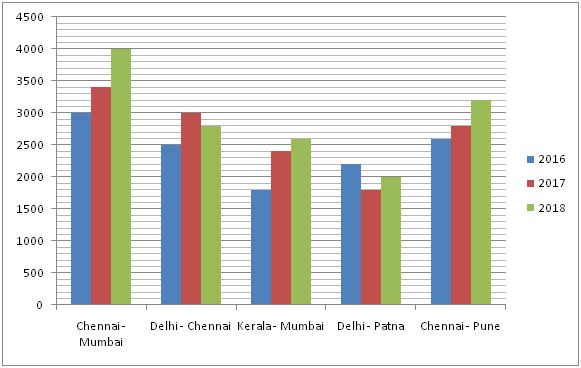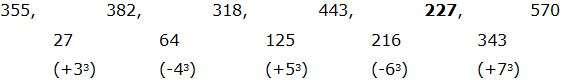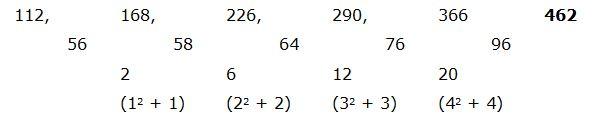# IBPS RRB Clerk/EPFO Assistant Prelims Quantitative Aptitude Questions 2019 (Day-11)

Dear Aspirants, Our IBPS Guide team is providing new series of Quantitative Aptitude Questions for IBPS RRB Clerk/EPFO Assistant Prelims 2019 so the aspirants can practice it on a daily basis. These questions are framed by our skilled experts after understanding your needs thoroughly. Aspirants can practice these new series questions daily to familiarize with the exact exam pattern and make your preparation effective.

### Click Here to Subscribe Crack High Level Puzzles & Seating Arrangement Questions PDF 2019 Plan

[WpProQuiz 6740]

Directions (Q. 1 – 5): What value should come in place of question mark (?) in the following number series?

1) 355, 382, 318, 443, ? , 570

a) 278

b) 312

c) 356

d) 227

e) 334

2) 56, 28, 42, 105, ? , 1653.75

a) 367.5

b) 255.5

c) 412.25

d) 433.75

e) 289.25

3) 12, 25, 84, 361, ? , 11205

a) 1568

b) 1672

c) 1854

d) 1744

e) 2056

4) 150, 934, 258, 834, 350, ?

a) 734

b) 750

c) 776

d) 712

e) 728

5) 112, 168, 226, 290, 366, ?

a) 546

b) 438

c) 570

d) 462

e) 524

Directions (Q. 6 – 10): Study the following information carefully and answer the given questions:

The following bar graph shows the total train fare of 5 different routes in 3 different years.6) Find the difference between the total train fare in the year 2016 to that of 2018 of all the given routes together?

a) 2500

b) 2800

c) 3200

d) 3500

e) None of these

7) Find the ratio between the total train fares of Delhi – Chennai to that of Chennai – Pune in all the given years together?

a) 67: 72

b) 125: 133

c) 219: 221

d) 83: 86

e) None of these

8) Total train fares in the year 2017 for the routes of Chennai – Mumbai, Kerala – Mumbai and Chennai – Pune together is approximately what percentage of total train fares in the year 2016 for the routes of Delhi – Chennai, Kerala – Mumbai and Delhi – Patna together?

a) 145 %

b) 132 %

c) 123 %

d) 106 %

e) 92 %

9) Find the average train fare in the year 2017 of all the given years together?

a) 2240

b) 2820

c) 3060

d) 2680

e) None of these

10) Total train fare in the year 2018 of all the given routes together is approximately what percentage more/less than the total train fare in the year 2017 of all the given routes together?

a) 15 % more

b) 9 % more

c) 20 % less

d) 9 % less

e) 20 % moreThe difference is, +33, -43, +53, -63, +73,…

The pattern is, *1/2, *3/2, *5/2, *7/2, *9/2,…

The pattern is, *2 + 12, *3 + 32, *4 + 52, *5 + 72, *6 + 92,…

The pattern is, +282, -262, +242, -222, +202,…The difference of difference is, 12 + 1, 22 + 2, 32 + 3, 42 + 4,…

(or)

The difference of difference is, (1*2), (2*3), (3*4), (4*5)

Direction (6-10) :

The total train fare in the year 2016 of all the given routes together

= > 3000 + 2500 + 1800 + 2200 + 2600 = 12100

The total train fare in the year 2018 of all the given routes together

= > 4000 + 2800 + 2600 + 2000 + 3200 = 14600

Required difference = 14600 – 12100 = 2500

The total train fares of Delhi – Chennai in all the given years together

= > 2500 + 3000 + 2800 = 8300

The total train fares of Chennai – Pune in all the given years together

= > 2600 + 2800 + 3200 = 8600

Required ratio = 8300: 8600 = 83: 86

Total train fares in the year 2017 for the routes of Chennai – Mumbai, Kerala – Mumbai and Chennai – Pune together

= > 3400 + 2400 + 2800 = 8600

Total train fares in the year 2016 for the routes of Delhi – Chennai, Kerala – Mumbai and Delhi – Patna together

= > 2500 + 1800 + 2200 = 6500

Required % = (8600/6500)*100 = 132 %

The average train fare in the year 2017 of all the given years together

= > (3400 + 3000 + 2400 + 1800 + 2800)/5

= > 13400/5 = 2680

Total train fare in the year 2018 of all the given routes together

= > 4000 + 2800 + 2600 + 2000 + 3200 = 14600

Total train fare in the year 2017 of all the given routes together

= > 3400 + 3000 + 2400 + 1800 + 2800 = 13400

Required % = [(14600 – 13400)/13400]*100 = 9 % more